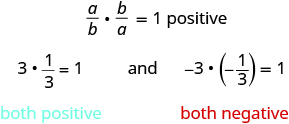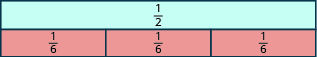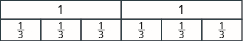$$\newcommand{\id}{\mathrm{id}}$$ $$\newcommand{\Span}{\mathrm{span}}$$ $$\newcommand{\kernel}{\mathrm{null}\,}$$ $$\newcommand{\range}{\mathrm{range}\,}$$ $$\newcommand{\RealPart}{\mathrm{Re}}$$ $$\newcommand{\ImaginaryPart}{\mathrm{Im}}$$ $$\newcommand{\Argument}{\mathrm{Arg}}$$ $$\newcommand{\norm}{\| #1 \|}$$ $$\newcommand{\inner}{\langle #1, #2 \rangle}$$ $$\newcommand{\Span}{\mathrm{span}}$$

# 4.4: Multiply and Divide Fractions (Part 2)

•• Contributed by OpenStax
• Mathematics at OpenStax CNX
$$\newcommand{\vecs}{\overset { \rightharpoonup} {\mathbf{#1}} }$$ $$\newcommand{\vecd}{\overset{-\!-\!\rightharpoonup}{\vphantom{a}\smash {#1}}}$$$$\newcommand{\id}{\mathrm{id}}$$ $$\newcommand{\Span}{\mathrm{span}}$$ $$\newcommand{\kernel}{\mathrm{null}\,}$$ $$\newcommand{\range}{\mathrm{range}\,}$$ $$\newcommand{\RealPart}{\mathrm{Re}}$$ $$\newcommand{\ImaginaryPart}{\mathrm{Im}}$$ $$\newcommand{\Argument}{\mathrm{Arg}}$$ $$\newcommand{\norm}{\| #1 \|}$$ $$\newcommand{\inner}{\langle #1, #2 \rangle}$$ $$\newcommand{\Span}{\mathrm{span}}$$ $$\newcommand{\id}{\mathrm{id}}$$ $$\newcommand{\Span}{\mathrm{span}}$$ $$\newcommand{\kernel}{\mathrm{null}\,}$$ $$\newcommand{\range}{\mathrm{range}\,}$$ $$\newcommand{\RealPart}{\mathrm{Re}}$$ $$\newcommand{\ImaginaryPart}{\mathrm{Im}}$$ $$\newcommand{\Argument}{\mathrm{Arg}}$$ $$\newcommand{\norm}{\| #1 \|}$$ $$\newcommand{\inner}{\langle #1, #2 \rangle}$$ $$\newcommand{\Span}{\mathrm{span}}$$

## Find Reciprocals

The fractions $$\dfrac{2}{3}$$ and $$\dfrac{3}{2}$$ are related to each other in a special way. So are $$− \dfrac{10}{7}$$ and $$− \dfrac{7}{10}$$. Do you see how? Besides looking like upside-down versions of one another, if we were to multiply these pairs of fractions, the product would be 1.

$\dfrac{2}{3} \cdot \dfrac{3}{2} = 1 \quad and \quad - \dfrac{10}{7} \left(- \dfrac{7}{10}\right) = 1 \tag{4.2.53} \nonumber$

Such pairs of numbers are called reciprocals.

Definition: Reciprocal

The reciprocal of the fraction $$\dfrac{a}{b}$$ is $$\dfrac{b}{a}$$, where $$a ≠ 0$$ and $$b ≠ 0$$.

A number and its reciprocal have a product of $$1$$.

$\dfrac{a}{b} \cdot \dfrac{b}{a} = 1 \tag{4.2.54}$

To find the reciprocal of a fraction, we invert the fraction. This means that we place the numerator in the denominator and the denominator in the numerator.

To get a positive result when multiplying two numbers, the numbers must have the same sign. So reciprocals must have the same sign.To find the reciprocal, keep the same sign and invert the fraction. The number zero does not have a reciprocal. Why? A number and its reciprocal multiply to $$1$$. Is there any number $$r$$ so that $$0 • r = 1$$? No. So, the number $$0$$ does not have a reciprocal.

Example $$\PageIndex{11}$$: reciprocal

Find the reciprocal of each number. Then check that the product of each number and its reciprocal is $$1$$.

1. $$\dfrac{4}{9}$$
2. $$− \dfrac{1}{6}$$
3. $$− \dfrac{14}{5}$$
4. $$7$$

Solution

To find the reciprocals, we keep the sign and invert the fractions.

1.
 Find the reciprocal of $$\dfrac{4}{9}$$. The reciprocal of $$\dfrac{4}{9}$$ is $$\dfrac{9}{4}$$.

Check:

 Multiply the number and its reciprocal. $$\dfrac{4}{9} \cdot \dfrac{9}{4}$$ Multiply numerators and denominators. $$\dfrac{36}{36}$$ Simplify. $$1 \; \checkmark$$
1.
 Find the reciprocal of $$- \dfrac{1}{6}$$. The reciprocal of $$- \dfrac{1}{6}$$ is $$\dfrac{6}{1}$$. Simplify. $$-6$$ Check. $$- \dfrac{1}{6} \cdot (-6) = 1 \; \checkmark$$
1.
 Find the reciprocal of $$- \dfrac{14}{5}$$. $$- \dfrac{5}{14}$$ Check. $$- \dfrac{14}{5} \cdot \left(- \dfrac{5}{14}\right) = \dfrac{70}{70} = 1 \; \checkmark$$
1.
 Find the reciprocal of 7. Write 7 as a fraction. $$\dfrac{7}{1}$$ Write the reciprocal of $$\dfrac{7}{1}$$. $$\dfrac{1}{7}$$ Check. $$7 \cdot \left(\dfrac{1}{7}\right) = 1 \; \checkmark$$

Exercise $$\PageIndex{21}$$

Find the reciprocal:

1. $$\dfrac{5}{7}$$
2. $$− \dfrac{1}{8}$$
3. $$− \dfrac{11}{4}$$
4. $$14$$

$$\dfrac{7}{5}$$

$$-8$$

$$-\dfrac{4}{11}$$

$$\dfrac{1}{14}$$

Exercise $$\PageIndex{22}$$

Find the reciprocal:

1. $$\dfrac{3}{7}$$
2. $$− \dfrac{1}{12}$$
3. $$− \dfrac{14}{9}$$
4. $$21$$

$$\dfrac{7}{3}$$

$$-12$$

$$-\dfrac{9}{14}$$

$$\dfrac{1}{21}$$

In a previous chapter, we worked with opposites and absolute values. Table $$\PageIndex{1}$$ compares opposites, absolute values, and reciprocals.

Table $$\PageIndex{1}$$
Opposite Absolute Value Reciprocal
has opposite sign is never negative has same sign, fraction inverts

Example $$\PageIndex{12}$$: fractions

Fill in the chart for each fraction in the left column:

Number Opposite Absolute Value Reciprocal
$$- \dfrac{3}{8}$$
$$\dfrac{1}{2}$$
$$\dfrac{9}{5}$$
$$-5$$

Solution

To find the opposite, change the sign. To find the absolute value, leave the positive numbers the same, but take the opposite of the negative numbers. To find the reciprocal, keep the sign the same and invert the fraction.

Number Opposite Absolute Value Reciprocal
$$- \dfrac{3}{8}$$ $$\dfrac{3}{8}$$ $$\dfrac{3}{8}$$ $$- \dfrac{8}{3}$$
$$\dfrac{1}{2}$$ $$- \dfrac{1}{2}$$ $$\dfrac{1}{2}$$ $$2$$
$$\dfrac{9}{5}$$ $$- \dfrac{9}{5}$$ $$\dfrac{9}{5}$$ $$\dfrac{5}{9}$$
$$-5$$ $$5$$ $$5$$ $$- \dfrac{1}{5}$$

Exercise $$\PageIndex{23}$$

Fill in the chart for each number given:

Number Opposite Absolute Value Reciprocal
$$- \dfrac{5}{8}$$
$$\dfrac{1}{4}$$
$$\dfrac{8}{3}$$
$$-8$$
Number Opposite Absolute Value Reciprocal
$$-\dfrac{5}{8}$$ $$\dfrac{5}{8}$$ $$\dfrac{5}{8}$$ $$-\dfrac{8}{5}$$
$$\dfrac{1}{4}$$ $$-\dfrac{1}{4}$$ $$\dfrac{1}{4}$$ $$4$$
$$\dfrac{8}{3}$$ $$-\dfrac{8}{3}$$ $$\dfrac{8}{3}$$ $$\dfrac{3}{8}$$
$$-8$$ $$8$$ $$8$$ $$-\dfrac{1}{8}$$

Exercise $$\PageIndex{24}$$

Fill in the chart for each number given:

Number Opposite Absolute Value Reciprocal
$$- \dfrac{4}{7}$$
$$\dfrac{1}{8}$$
$$\dfrac{9}{4}$$
$$-1$$
Number Opposite Absolute Value Reciprocal
$$-\dfrac{4}{7}$$ $$\dfrac{4}{7}$$ $$\dfrac{4}{7}$$ $$- \dfrac{7}{4}$$
$$\dfrac{1}{8}$$ $$-\dfrac{1}{8}$$ $$\dfrac{1}{8}$$ $$8$$
$$\dfrac{9}{4}$$ $$-\dfrac{9}{4}$$ $$\dfrac{9}{4}$$ $$\dfrac{4}{9}$$
$$-1$$ $$1$$ $$1$$ $$-\dfrac{1}{1}$$

## Divide Fractions

Why is $$12 ÷ 3 = 4$$? We previously modeled this with counters. How many groups of $$3$$ counters can be made from a group of $$12$$ counters?Figure $$\PageIndex{2}$$

There are $$4$$ groups of $$3$$ counters. In other words, there are four $$3$$s in $$12$$. So, $$12 ÷ 3 = 4$$.

What about dividing fractions? Suppose we want to find the quotient: $$\dfrac{1}{2} \div \dfrac{1}{6}$$. We need to figure out how many $$\dfrac{1}{6}$$s there are in $$\dfrac{1}{2}$$. We can use fraction tiles to model this division. We start by lining up the half and sixth fraction tiles as shown in Figure $$\PageIndex{3}$$. Notice, there are three $$\dfrac{1}{6}$$ tiles in $$\dfrac{1}{2}$$, so $$\dfrac{1}{2} \div \dfrac{1}{6} = 3$$.Figure $$\PageIndex{3}$$

Example $$\PageIndex{13}$$: model

Model: $$\dfrac{1}{4} \div \dfrac{1}{8}$$.

Solution

We want to determine how many $$\dfrac{1}{8}$$s are in $$\dfrac{1}{4}$$. Start with one $$\dfrac{1}{4}$$ tile. Line up $$\dfrac{1}{8}$$ tiles underneath the $$\dfrac{1}{4}$$ tile.

Exercise $$\PageIndex{25}$$

Model: $$\dfrac{1}{3} \div \dfrac{1}{6}$$.Exercise $$\PageIndex{26}$$

Model: $$\dfrac{1}{2} \div \dfrac{1}{4}$$.Example $$\PageIndex{14}$$: model

Model: $$2 ÷ \dfrac{1}{4}$$.

Solution

We are trying to determine how many $$\dfrac{1}{4}$$s there are in $$2$$. We can model this as shown.Because there are eight $$\dfrac{1}{4}$$s in $$2$$, $$2 ÷ \dfrac{1}{4} = 8$$.

Exercise $$\PageIndex{27}$$

Model: $$2 ÷ \dfrac{1}{3}$$Exercise $$\PageIndex{28}$$

Model: $$3 ÷ \dfrac{1}{2}$$Let’s use money to model $$2 ÷ \dfrac{1}{4}$$ in another way. We often read $$\dfrac{1}{4}$$ as a ‘quarter’, and we know that a quarter is one-fourth of a dollar as shown in Figure $$\PageIndex{4}$$. So we can think of $$2 ÷ \dfrac{1}{4}$$ as, “How many quarters are there in two dollars?” One dollar is $$4$$ quarters, so $$2$$ dollars would be $$8$$ quarters. So again, $$2 ÷ \dfrac{1}{4} = 8$$.Figure $$\PageIndex{4}$$:The U.S. coin called a quarter is worth one-fourth of a dollar.

Using fraction tiles, we showed that $$\dfrac{1}{2} \div \dfrac{1}{6} = 3$$. Notice that $$\dfrac{1}{2} \cdot \dfrac{6}{1} = 3$$ also. How are $$\dfrac{1}{6}$$ and $$\dfrac{6}{1}$$ related? They are reciprocals. This leads us to the procedure for fraction division.

Definition: Fraction Division

If $$a, b, c,$$ and $$d$$ are numbers where $$b ≠ 0$$, $$c ≠ 0$$, and $$d ≠ 0$$, then

$\dfrac{a}{b} \div \dfrac{c}{d} = \dfrac{a}{b} \cdot \dfrac{d}{c}$

To divide fractions, multiply the first fraction by the reciprocal of the second.

We need to say $$b ≠ 0$$, $$c ≠ 0$$ and $$d ≠ 0$$ to be sure we don’t divide by zero.

Example $$\PageIndex{15}$$: divide

Divide, and write the answer in simplified form: $$\dfrac{2}{5} \div \left(- \dfrac{3}{7}\right). Solution  Multiply the first fraction by the reciprocal of the second. \(\dfrac{2}{5} \left(- \dfrac{7}{3}\right)$$ Multiply. The product is negative. $$- \dfrac{14}{15}$$

Exercise $$\PageIndex{29}$$

Divide, and write the answer in simplified form: $$\dfrac{3}{7} \div \left(− \dfrac{2}{3}\right)$$.

$$-\dfrac{9}{14}$$

Exercise $$\PageIndex{30}$$

Divide, and write the answer in simplified form: $$\dfrac{2}{3} \div \left(− \dfrac{7}{5}\right)$$.

$$-\dfrac{10}{21}$$

Example $$\PageIndex{16}$$: divide

Divide, and write the answer in simplified form: $$\dfrac{2}{3} \div \dfrac{n}{5}$$.

Solution

 Multiply the first fraction by the reciprocal of the second. $$\dfrac{2}{3} \div \dfrac{5}{n}$$ Multiply. $$\dfrac{10}{3n}$$

Exercise $$\PageIndex{31}$$

Divide, and write the answer in simplified form: $$\dfrac{3}{5} \div \dfrac{p}{7}$$.

$$\dfrac{21}{5p}$$

Exercise $$\PageIndex{32}$$

Divide, and write the answer in simplified form: $$\dfrac{5}{8} \div \dfrac{q}{3}$$.

$$\dfrac{15}{8q}$$

Example $$\PageIndex{17}$$: divide

Divide, and write the answer in simplified form: $$− \dfrac{3}{4} \div \left(− \dfrac{7}{8}\right)$$.

Solution

 Multiply the first fraction by the reciprocal of the second. $$- \dfrac{3}{4} \cdot \left(- \dfrac{8}{7}\right)$$ Multiply. Remember to determine the sign first. $$\dfrac{3 \cdot 8}{4 \cdot 7}$$ Rewrite to show common factors. $$\dfrac{3 \cdot \cancel{4} \cdot 2}{\cancel{4} \cdot 7}$$ Remove common factors and simplify. $$\dfrac{6}{7}$$

Exercise $$\PageIndex{33}$$

Divide, and write the answer in simplified form: $$− \dfrac{2}{3} \div \left(− \dfrac{5}{6}\right)$$.

$$\dfrac{4}{5}$$

Exercise $$\PageIndex{34}$$

Divide, and write the answer in simplified form: $$− \dfrac{5}{6} \div \left(− \dfrac{2}{3}\right)$$.

$$\dfrac{5}{4}$$

Example $$\PageIndex{18}$$: divide

Divide, and write the answer in simplified form: $$\dfrac{7}{18} \div \dfrac{14}{27}$$.

Solution

 Multiply the first fraction by the reciprocal of the second. $$\dfrac{7}{18} \cdot \dfrac{27}{14}$$ Multiply. $$\dfrac{7 \cdot 27}{18 \cdot 14}$$ Rewrite showing common factors. $$\dfrac{\cancel{\textcolor{red}{7}} \cdot \cancel{\textcolor{red}{9}} \cdot 3}{\cancel{\textcolor{red}{9}} \cdot \cancel{\textcolor{red}{7}} \cdot 2}$$ Remove common factors. $$\dfrac{3}{2 \cdot 2}$$ Simplify. $$\dfrac{3}{4}$$

Exercise $$\PageIndex{35}$$

Divide, and write the answer in simplified form: $$\dfrac{7}{27} \div \dfrac{35}{36}$$.

$$\dfrac{4}{15}$$

Exercise $$\PageIndex{36}$$

Divide, and write the answer in simplified form: $$\dfrac{5}{14} \div \dfrac{15}{28}$$.

$$\dfrac{2}{3}$$

## Key Concepts

• Equivalent Fractions Property
• If $$a, b, c$$ are numbers where $$b\neq 0, c\neq 0$$, then $$\dfrac{a}{b} = \dfrac{a\cdot c}{b\cdot c}$$ and $$\dfrac{a\cdot c}{b\cdot c} = \dfrac{a}{b}$$
• Simplify a fraction.
1. Rewrite the numerator and denominator to show the common factors. If needed, factor the numerator and denominator into prime numbers.
2. Simplify, using the equivalent fractions property, by removing common factors.
3. Multiply any remaining factors.
• Fraction Multiplication
• If $$a, b, c,$$ and
• Reciprocal
• A number and its reciprocal have a product of 1. $$\frac{a}{b} \cdot \frac{b}{a} = 1$$
•  Opposite Absolute Value Reciprocal has opposite sign is never negative has same sign, fraction inverts
• Fraction Division
• If $$a, b, c,$$ and $$d$$ are numbers where $$b\neq 0$$, $$c\neq 0$$, and $$d\neq 0$$, then $$\dfrac{a}{b} \div \dfrac{c}{d} = \dfrac{a}{b}\cdot \dfrac{d}{c}$$
• To divide fractions, multiply the first fraction by the reciprocal of the second.

## Glossary

reciprocal

The reciprocal of the fraction $$\dfrac{a}{b}$$ is $$\dfrac{b}{a}$$ where $$a\neq 0$$ and $$b\neq 0$$.

simplified fraction

A fraction is considered simplified if there are no common factors in the numerator and denominator.

## Practice Makes Perfect

### Simplify Fractions

In the following exercises, simplify each fraction. Do not convert any improper fractions to mixed numbers.

1. $$\dfrac{7}{21}$$
2. $$\dfrac{8}{24}$$
3. $$\dfrac{15}{20}$$
4. $$\dfrac{12}{18}$$
5. $$- \dfrac{40}{88}$$
6. $$- \dfrac{63}{99}$$
7. $$- \dfrac{108}{63}$$
8. $$- \dfrac{104}{48}$$
9. $$\dfrac{120}{252}$$
10. $$\dfrac{182}{294}$$
11. $$- \dfrac{168}{192}$$
12. $$- \dfrac{140}{224}$$
13. $$\dfrac{11x}{11y}$$
14. $$\dfrac{15a}{15b}$$
15. $$− \dfrac{3x}{12y}$$
16. $$− \dfrac{4x}{32y}$$
17. $$\dfrac{14x^{2}}{21y}$$
18. $$\dfrac{24a}{32b^{2}}$$

### Multiply Fractions

In the following exercises, use a diagram to model.

1. $$\dfrac{1}{2} \cdot \dfrac{2}{3}$$
2. $$\dfrac{1}{2} \cdot \dfrac{5}{8}$$
3. $$\dfrac{1}{3} \cdot \dfrac{5}{6}$$
4. $$\dfrac{1}{3} \cdot \dfrac{2}{5}$$

In the following exercises, multiply, and write the answer in simplified form.

1. $$\dfrac{2}{5} \cdot \dfrac{1}{3}$$
2. $$\dfrac{1}{2} \cdot \dfrac{3}{8}$$
3. $$\dfrac{3}{4} \cdot \dfrac{9}{10}$$
4. $$\dfrac{4}{5} \cdot \dfrac{2}{7}$$
5. $$− \dfrac{2}{3} \left(− \dfrac{3}{8}\right)$$
6. $$− \dfrac{3}{4} \left(− \dfrac{4}{9}\right)$$
7. $$- \dfrac{5}{9} \cdot \dfrac{3}{10}$$
8. $$- \dfrac{3}{8} \cdot \dfrac{4}{15}$$
9. $$− \dfrac{7}{12} \left(− \dfrac{8}{21}\right)$$
10. $$\dfrac{5}{12} \left(− \dfrac{8}{15}\right)$$
11. $$\left(− \dfrac{14}{15}\right) \left(\dfrac{9}{20}\right)$$
12. $$\left(− \dfrac{9}{10}\right) \left(\dfrac{25}{33}\right)$$
13. $$\left(− \dfrac{63}{84}\right) \left(- \dfrac{44}{90}\right)$$
14. $$\left(− \dfrac{33}{60}\right) \left(- \dfrac{40}{88}\right)$$
15. $$4 \cdot \dfrac{5}{11}$$
16. $$5 \cdot \dfrac{8}{3}$$
17. $$\dfrac{3}{7} \cdot 21n$$
18. $$\dfrac{5}{6} \cdot 30m$$
19. $$−28p \left(− \dfrac{1}{4}\right)$$
20. $$−51q \left(− \dfrac{1}{3}\right)$$
21. $$−8 \left(\dfrac{17}{4}\right)$$
22. $$\dfrac{14}{5} (−15)$$
23. $$−1 \left(− \dfrac{3}{8}\right)$$
24. $$(−1) \left(- \dfrac{6}{7}\right)$$
25. $$\left(\dfrac{2}{3}\right)^{3}$$
26. $$\left(\dfrac{4}{5}\right)^{2}$$
27. $$\left(\dfrac{6}{5}\right)^{4}$$
28. $$\left(\dfrac{4}{7}\right)^{4}$$

Find Reciprocals In the following exercises, find the reciprocal.

1. $$\dfrac{3}{4}$$
2. $$\dfrac{2}{3}$$
3. $$− \dfrac{5}{17}$$
4. $$− \dfrac{6}{19}$$
5. $$\dfrac{11}{8}$$
6. −13
7. −19
8. −1
9. 1
10. Fill in the chart.
Opposite Absolute Value Reciprocal
$$- \dfrac{7}{11}$$
$$\dfrac{4}{5}$$
$$\dfrac{10}{7}$$
$$-8$$
11. Fill in the chart.
Opposite Absolute Value Reciprocal
$$- \dfrac{3}{13}$$
$$\dfrac{9}{14}$$
$$\dfrac{15}{7}$$
$$-9$$

#### Divide Fractions

In the following exercises, model each fraction division.

1. $$\dfrac{1}{2} \div \dfrac{1}{4}$$
2. $$\dfrac{1}{2} \div \dfrac{1}{8}$$
3. $$2 \div \dfrac{1}{5}$$
4. $$3 \div \dfrac{1}{4}$$

In the following exercises, divide, and write the answer in simplified form.

1. $$\dfrac{1}{2} \div \dfrac{1}{4}$$
2. $$\dfrac{1}{2} \div \dfrac{1}{8}$$
3. $$\dfrac{3}{4} \div \dfrac{2}{3}$$
4. $$\dfrac{4}{5} \div \dfrac{3}{4}$$
5. $$- \dfrac{4}{5} \div \dfrac{4}{7}$$
6. $$- \dfrac{3}{4} \div \dfrac{3}{5}$$
7. $$− \dfrac{7}{9} \div \left(- \dfrac{7}{9}\right)$$
8. $$− \dfrac{5}{6} \div \left(- \dfrac{5}{6}\right)$$
9. $$\dfrac{3}{4} \div \dfrac{x}{11}$$
10. $$\dfrac{2}{5} \div \dfrac{y}{9}$$
11. $$\dfrac{5}{8} \div \dfrac{a}{10}$$
12. $$\dfrac{5}{6} \div \dfrac{c}{15}$$
13. $$\dfrac{5}{18} \div \left(- \dfrac{15}{24}\right)$$
14. $$\dfrac{7}{18} \div \left(- \dfrac{14}{27}\right)$$
15. $$\dfrac{7p}{12} \div \dfrac{21p}{8}$$
16. $$\dfrac{5q}{12} \div \dfrac{15q}{8}$$
17. $$\dfrac{8u}{15} \div \dfrac{12v}{25}$$
18. $$\dfrac{12r}{25} \div \dfrac{18s}{35}$$
19. $$-5 \div \dfrac{1}{2}$$
20. $$-3 \div \dfrac{1}{4}$$
21. $$\dfrac{3}{4} \div (-12)$$
22. $$\dfrac{2}{5} \div (-10)$$
23. $$−18 \div \left(− \dfrac{9}{2}\right)$$
24. $$−15 \div \left(− \dfrac{5}{3}\right)$$
25. $$\dfrac{1}{2} \div \left(- \dfrac{3}{4}\right) \div \dfrac{7}{8}$$
26. $$\dfrac{11}{2} \div \dfrac{7}{8} \cdot \dfrac{2}{11}$$

## Everyday Math

1. Baking A recipe for chocolate chip cookies calls for 3 4 cup brown sugar. Imelda wants to double the recipe.
1. How much brown sugar will Imelda need? Show your calculation. Write your result as an improper fraction and as a mixed number.
2. Measuring cups usually come in sets of $$\dfrac{1}{8}, \dfrac{1}{4}, \dfrac{1}{3}, \dfrac{1}{2}$$, and 1 cup. Draw a diagram to show two different ways that Imelda could measure the brown sugar needed to double the recipe.
2. Baking Nina is making 4 pans of fudge to serve after a music recital. For each pan, she needs 2 3 cup of condensed milk.
1. How much condensed milk will Nina need? Show your calculation. Write your result as an improper fraction and as a mixed number.
2. Measuring cups usually come in sets of $$\dfrac{1}{8}, \dfrac{1}{4}, \dfrac{1}{3}, \dfrac{1}{2}$$, and 1 cup. Draw a diagram to show two different ways that Nina could measure the condensed milk she needs.
3. Portions Don purchased a bulk package of candy that weighs 5 pounds. He wants to sell the candy in little bags that hold $$\dfrac{1}{4}$$ pound. How many little bags of candy can he fill from the bulk package?
4. Portions Kristen has $$\dfrac{3}{4}$$ yards of ribbon. She wants to cut it into equal parts to make hair ribbons for her daughter’s 6 dolls. How long will each doll’s hair ribbon be?

## Writing Exercises

1. Explain how you find the reciprocal of a fraction.
2. Explain how you find the reciprocal of a negative fraction.
3. Rafael wanted to order half a medium pizza at a restaurant. The waiter told him that a medium pizza could be cut into 6 or 8 slices. Would he prefer 3 out of 6 slices or 4 out of 8 slices? Rafael replied that since he wasn’t very hungry, he would prefer 3 out of 6 slices. Explain what is wrong with Rafael’s reasoning.
4. Give an example from everyday life that demonstrates how $$\dfrac{1}{2} \cdot \dfrac{2}{3}$$ is $$\dfrac{1}{3}$$.

## Self Check

(a) After completing the exercises, use this checklist to evaluate your mastery of the objectives of this section.(b) After reviewing this checklist, what will you do to become confident for all objectives?